# Wallpaper

Written by Paul Bourke
January 1991
Images updated January 2018
xn+1 = yn - sign(xn) | b xn - c |1/2
yn+1 = a - xna = 1, b = 4, c = 60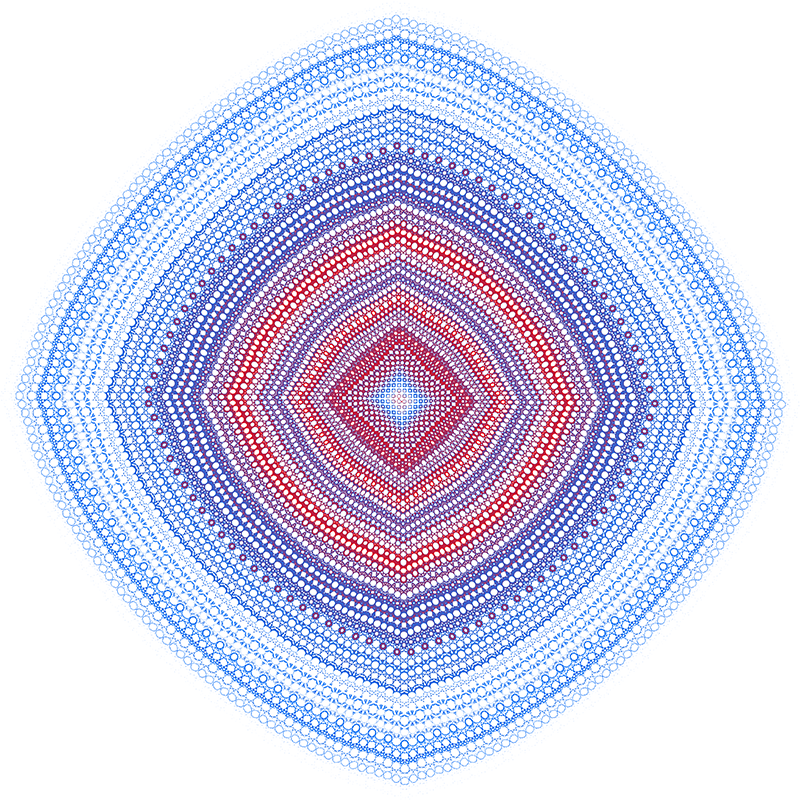a = 2, b = -1, c = 200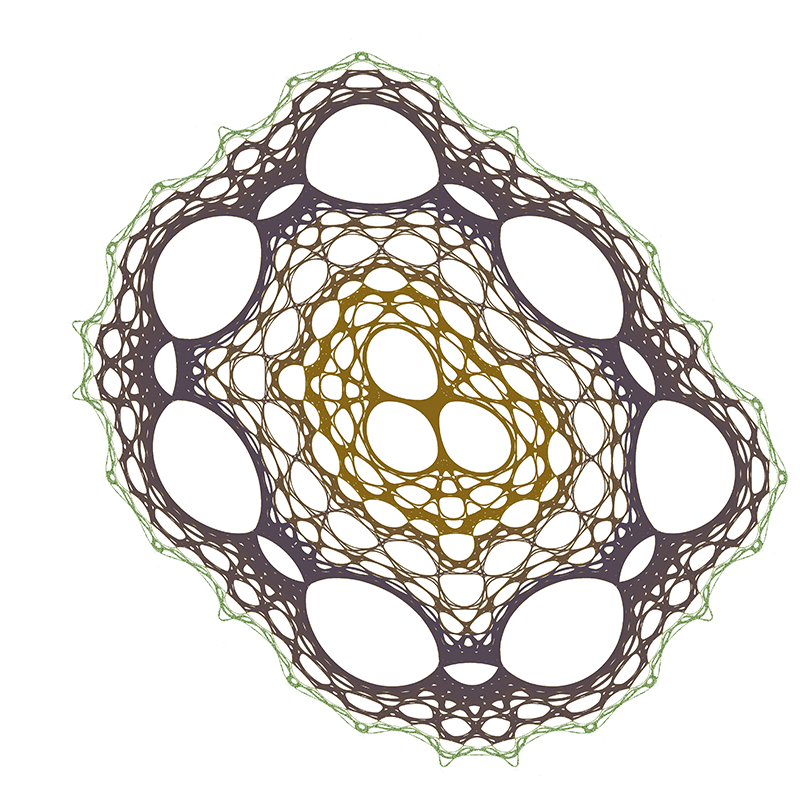a = 10, b = -10, c = 0.01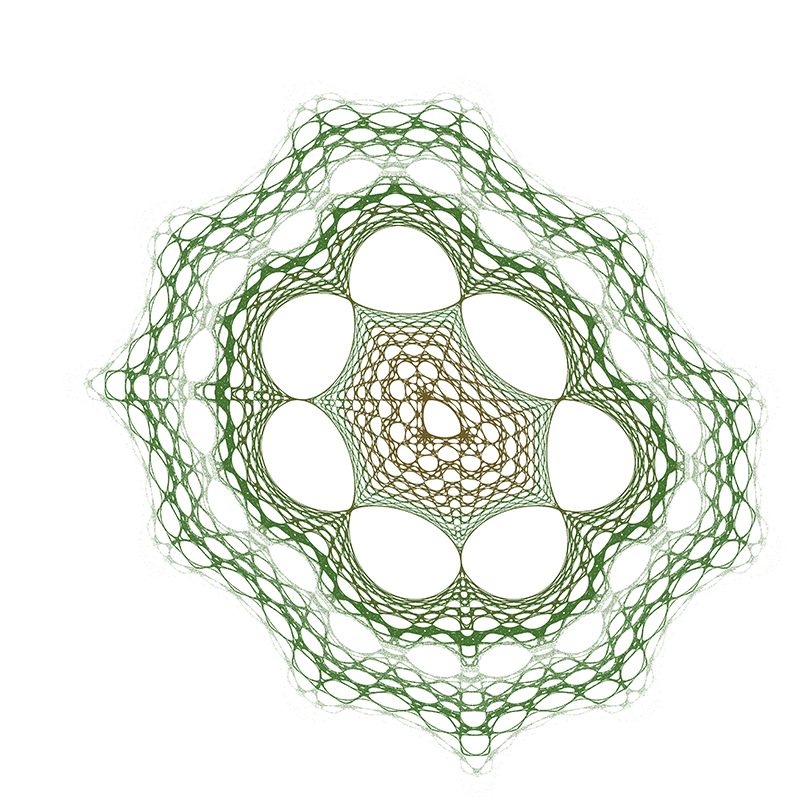a = 31, b = 12, c = 0.5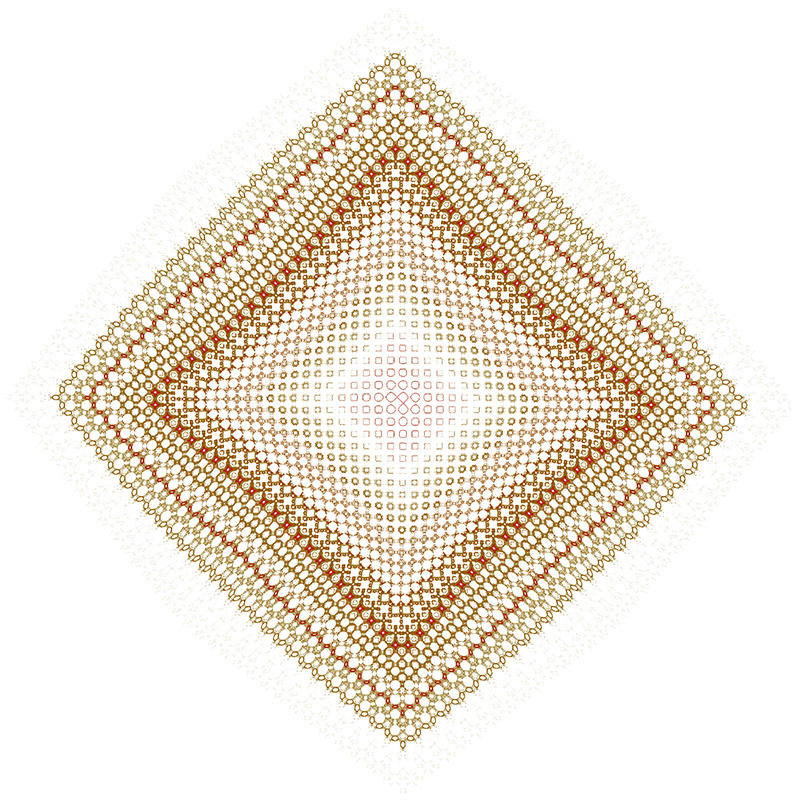a = 0.1, b = 0.1, c = 10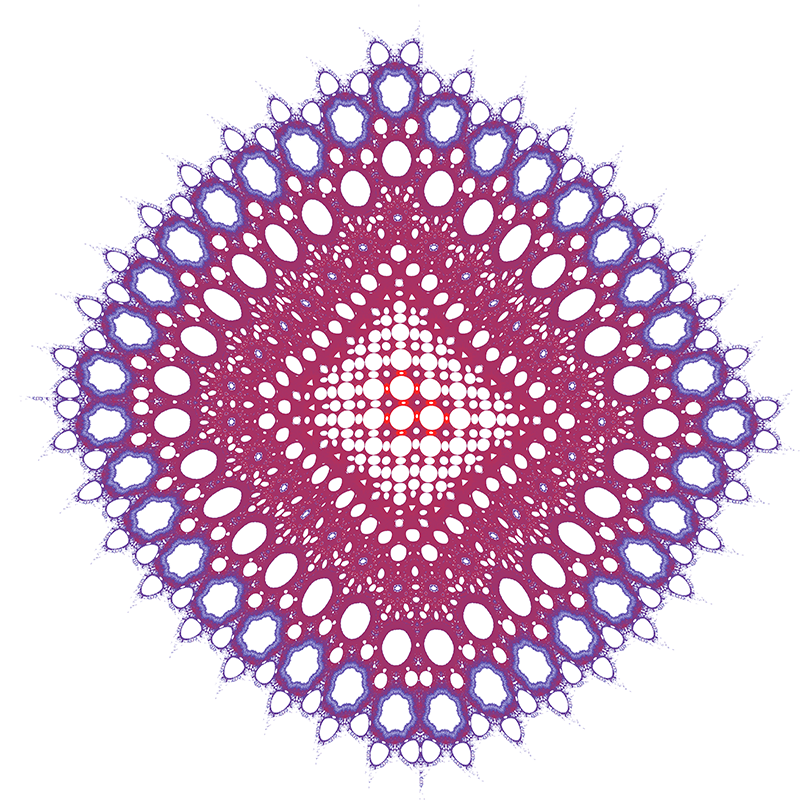a = 10, b = 1, c = 60